# 【算法】五分钟算法小知识：洗牌算法-问答-阿里云开发者社区-阿里云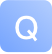## 【算法】五分钟算法小知识：洗牌算法

``````// 得到一个在闭区间 [min, max] 内的随机整数
int randInt(int min, int max);

// 第一种写法
void shuffle(int[] arr) {
int n = arr.length();
/******** 区别只有这两行 ********/
for (int i = 0 ; i < n; i++) {
// 从 i 到最后随机选一个元素
int rand = randInt(i, n - 1);
/*************************/
swap(arr[i], arr[rand]);
}
}

// 第二种写法
for (int i = 0 ; i < n - 1; i++)
int rand = randInt(i, n - 1);

// 第三种写法
for (int i = n - 1 ; i >= 0; i--)
int rand = randInt(0, i);

// 第四种写法
for (int i = n - 1 ; i > 0; i--)
int rand = randInt(0, i);
``````

``````// 假设传入这样一个 arr
int[] arr = {1,3,5,7,9};

void shuffle(int[] arr) {
int n = arr.length(); // 5
for (int i = 0 ; i < n; i++) {
int rand = randInt(i, n - 1);
swap(arr[i], arr[rand]);
}
}
``````

for 循环第一轮迭代时，i=0，rand 的取值范围是 [0,4]，有 5 个可能的取值。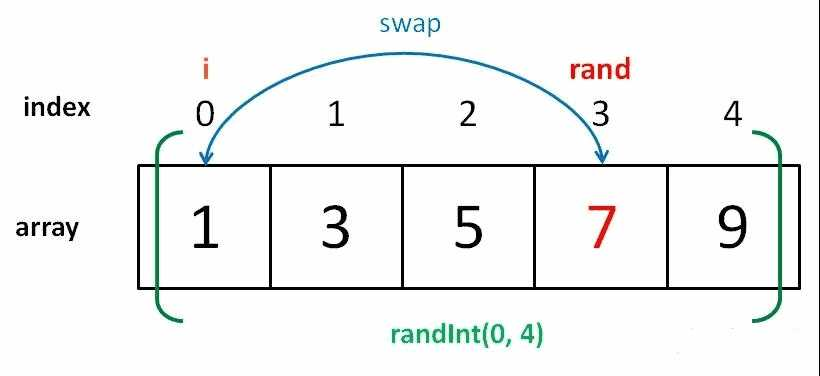for 循环第二轮迭代时，i=1，rand 的取值范围是 [1,4]，有 4 个可能的取值。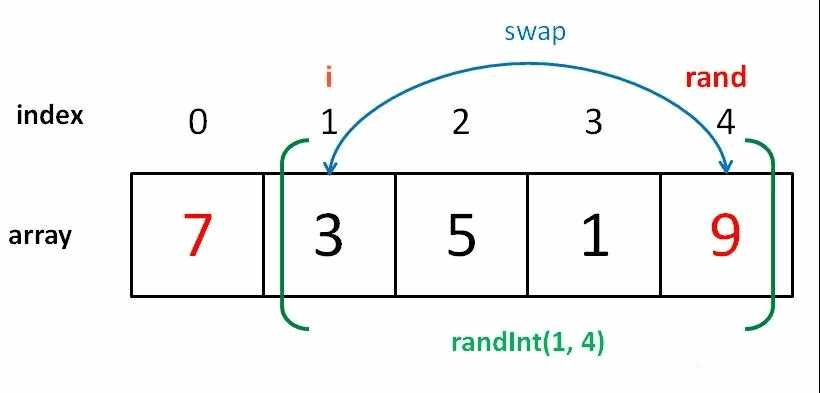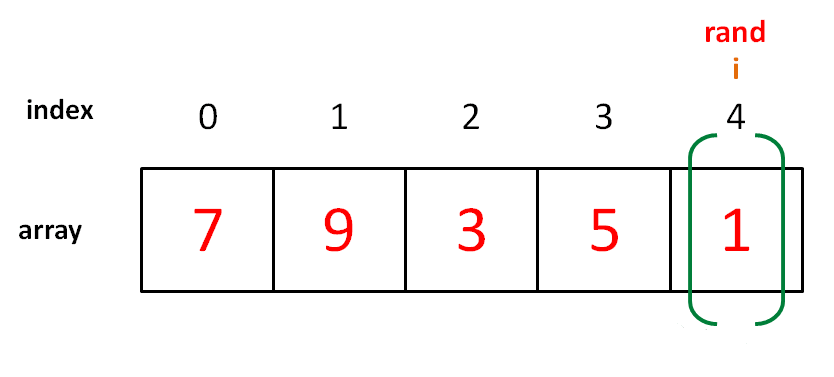``````// 第二种写法
// arr = {1,3,5,7,9}, n = 5
for (int i = 0 ; i < n - 1; i++)
int rand = randInt(i, n - 1);
``````

``````void shuffle(int[] arr) {
int n = arr.length();
for (int i = 0 ; i < n; i++) {
// 每次都从闭区间 [0, n-1]
// 中随机选取元素进行交换
int rand = randInt(0, n - 1);
swap(arr[i], arr[rand]);
}
}
``````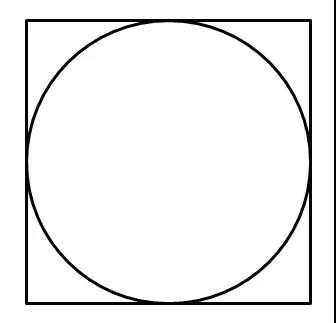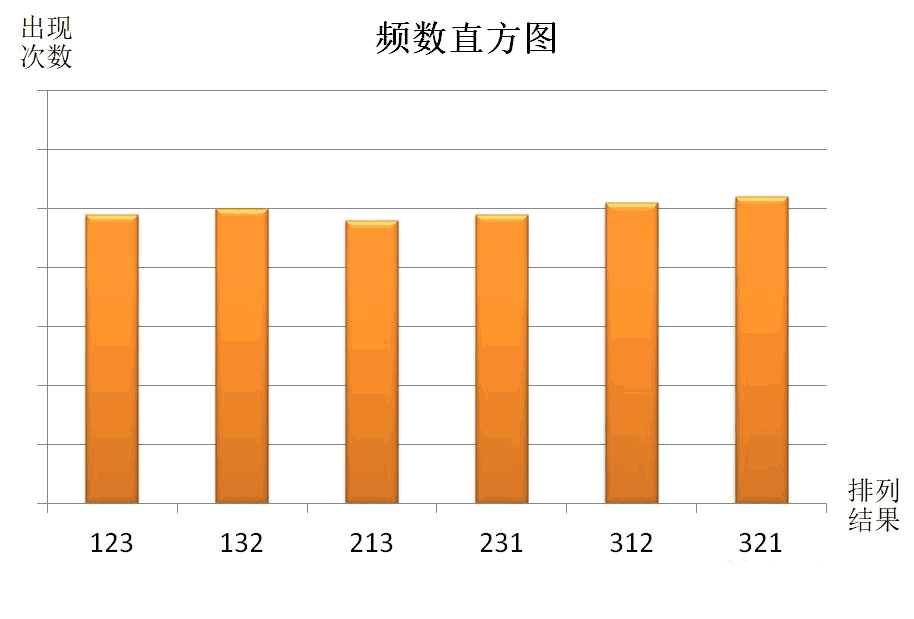``````void shuffle(int[] arr);

// 蒙特卡罗
int N = 1000000;
HashMap count; // 作为直方图
for (i = 0; i < N; i++) {
int[] arr = {1,2,3};
shuffle(arr);
// 此时 arr 已被打乱
count[arr] += 1；
}
for (int feq : count.values())
print(feq / N + " "); // 频率
``````

``````void shuffle(int[] arr);

// 蒙特卡罗方法
int N = 1000000;
int[] arr = {1,0,0,0,0};
int[] count = new int[arr.length];
for (int i = 0; i < N; i++) {
shuffle(arr); // 打乱 arr
for (int j = 0; j < arr.length; j++)
if (arr[j] == 1) {
count[j]++;
break;
}
}
for (int feq : count)
print(feq / N + " "); // 频率
``````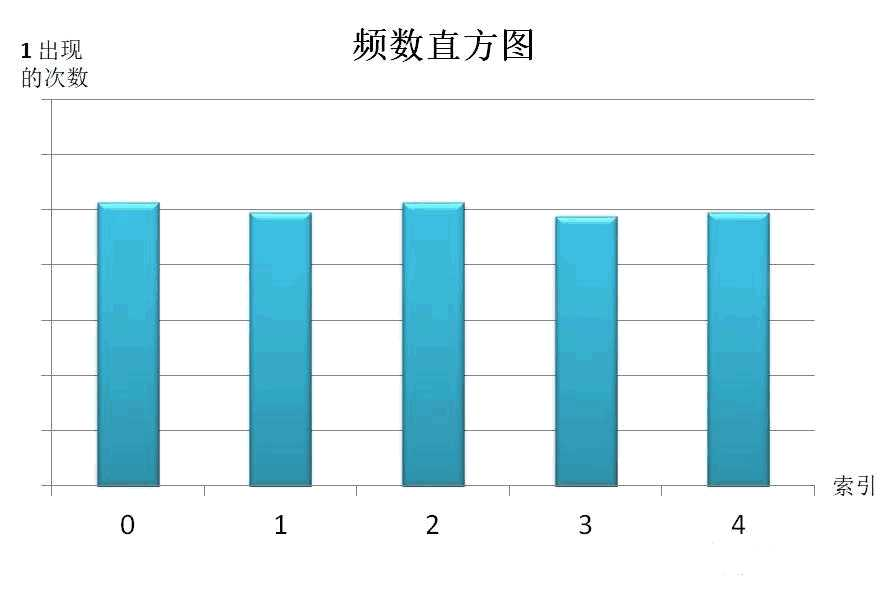1 条回答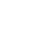写回答取消 提交回答
• 收藏

2020-05-06 14:22:06
赞同 展开评论 打赏

805
1
0
600
1
0
436
1
0
249
1
0
528
1
0
655
1
0
496
1
0
1879
0
0
2971
1
0
2324
1
0

【算法实战】2. K近邻算法1545

【算法实战】10. 树回归算法315

【算法实战】5. Logistic回归算法476

【算法实战】4. 朴素贝叶斯算法1156

【算法实战】9. 线性回归算法405

【算法实战】6. 支持向量机算法6210